### Special Relativity

After establishing that light travels with a finite speed and discovering Fraunhofer diffraction, $19^\text{th}$-century scientists had every reason to believe that light was a wave. Like sound traveling through air, or shock waves through Earth's crust, light appeared to be a disturbance in a medium it was travelling through. They called this medium luminiferous aether, which was thought to permeate all of space.Proponents of aether theory had been inspired by Rømer's work centuries earlier. They reasoned that Earth moved relative to the aether as Earth orbited the Sun. Consequently, they hoped to deduce aether's properties by observing changes in the light from distant stars throughout the year.

In this quiz, we will first look at how observers moving relative to a medium detect waves. Then we will extend our insights to light moving through the mysterious luminiferous aether.

# The Mystery of the Luminiferous Aether

Imagine a submarine traveling through a long tank of water. You already know from experience that if we want the submarine to move faster, we can push on it with greater force, independent of the water it moves through.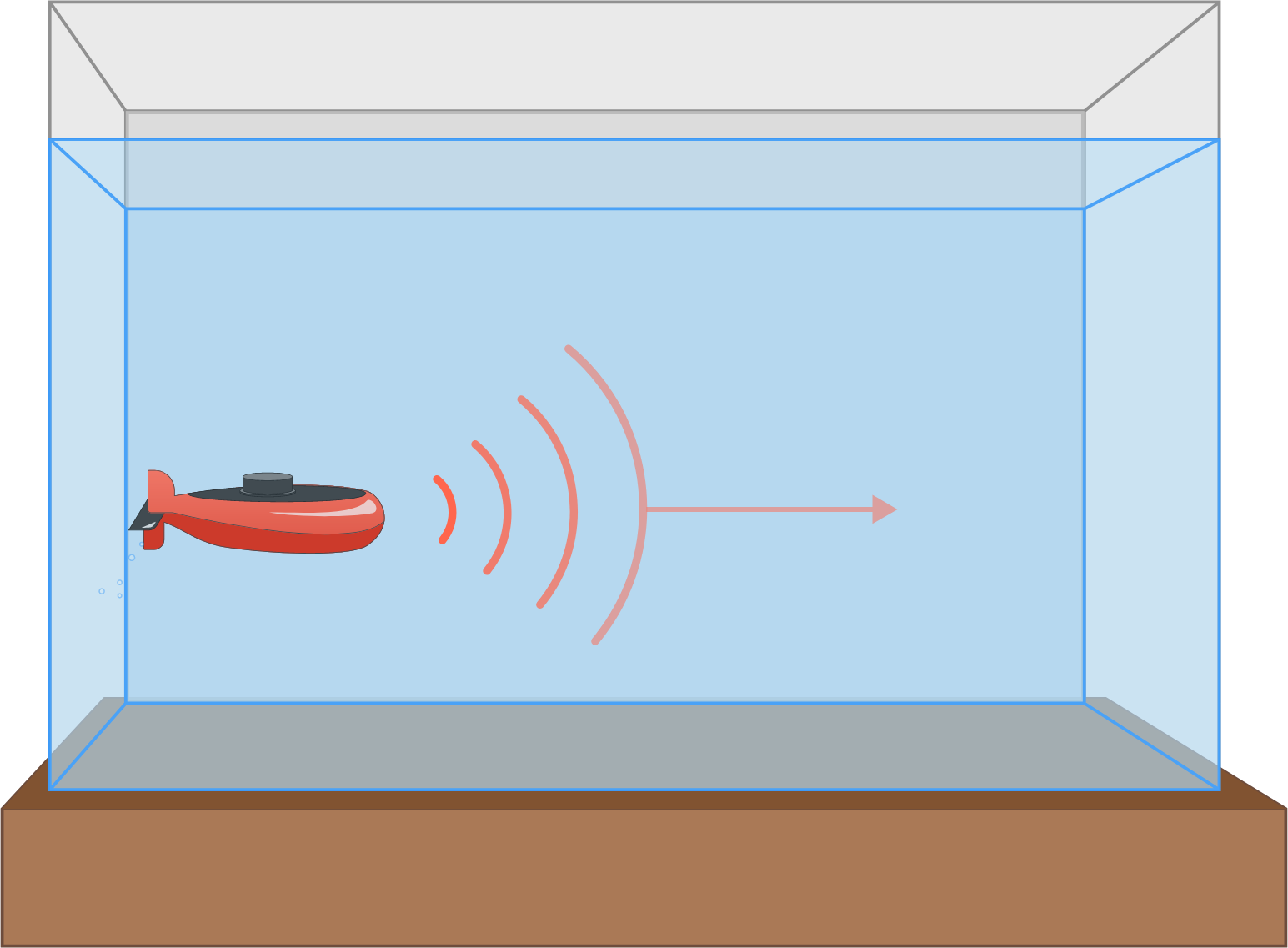However, propagating waves in water are different. They are small changes in water pressure that move along at the speed of sound. They are not material objects like the submarine.

Consider the sound waves generated by a sonar transmitter in the submarine's nose. How can we make the sound waves travel faster with respect to the ground?

# The Mystery of the Luminiferous Aether

Nineteenth-century scientists called the medium that light propagates through luminiferous aether.

The problem with this wave model of light was that no one could say for sure what the aether was. An obvious question they asked to get to the bottom of this mystery is, “At what speed is the luminiferous aether traveling?" or rather, "What speed are we traveling at relative to it?”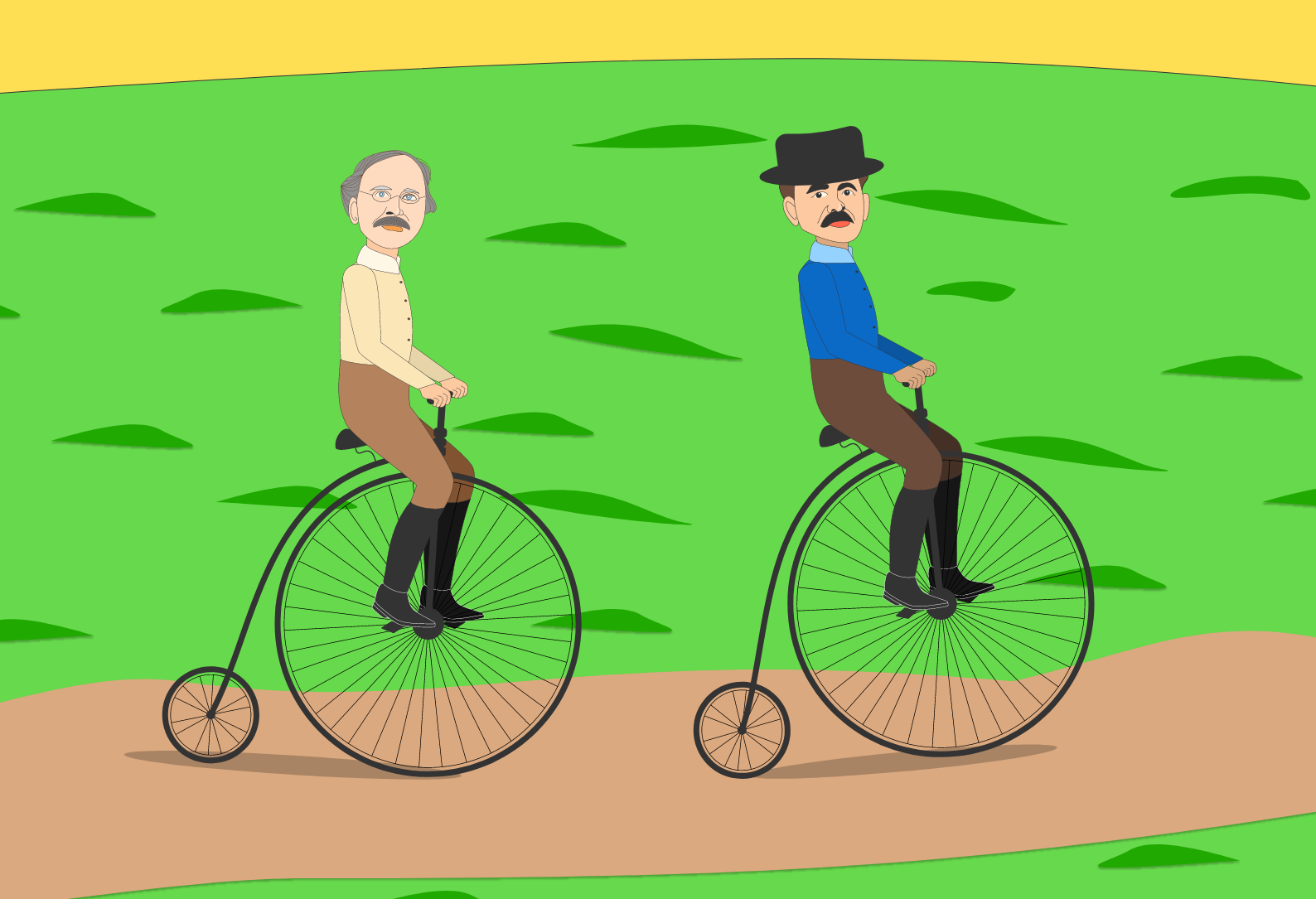In the $1880$s, experimentalists Albert Michelson and Edward Morley set out to answer this question. Their idea was simple: if Earth is moving with respect to the aether, then we should be able to detect an asymmetry in how long it takes to send a light signal one way versus another.

Let's illustrate with a thought experiment... on bikes.

# The Mystery of the Luminiferous Aether

Even when he's on a lazy bike ride, Michelson's mind is never liberated from plans of his next experiment, and he's about to collide with a tree. Morley calls out a warning from a distance of $L_0$ behind Michelson, but it might be too late.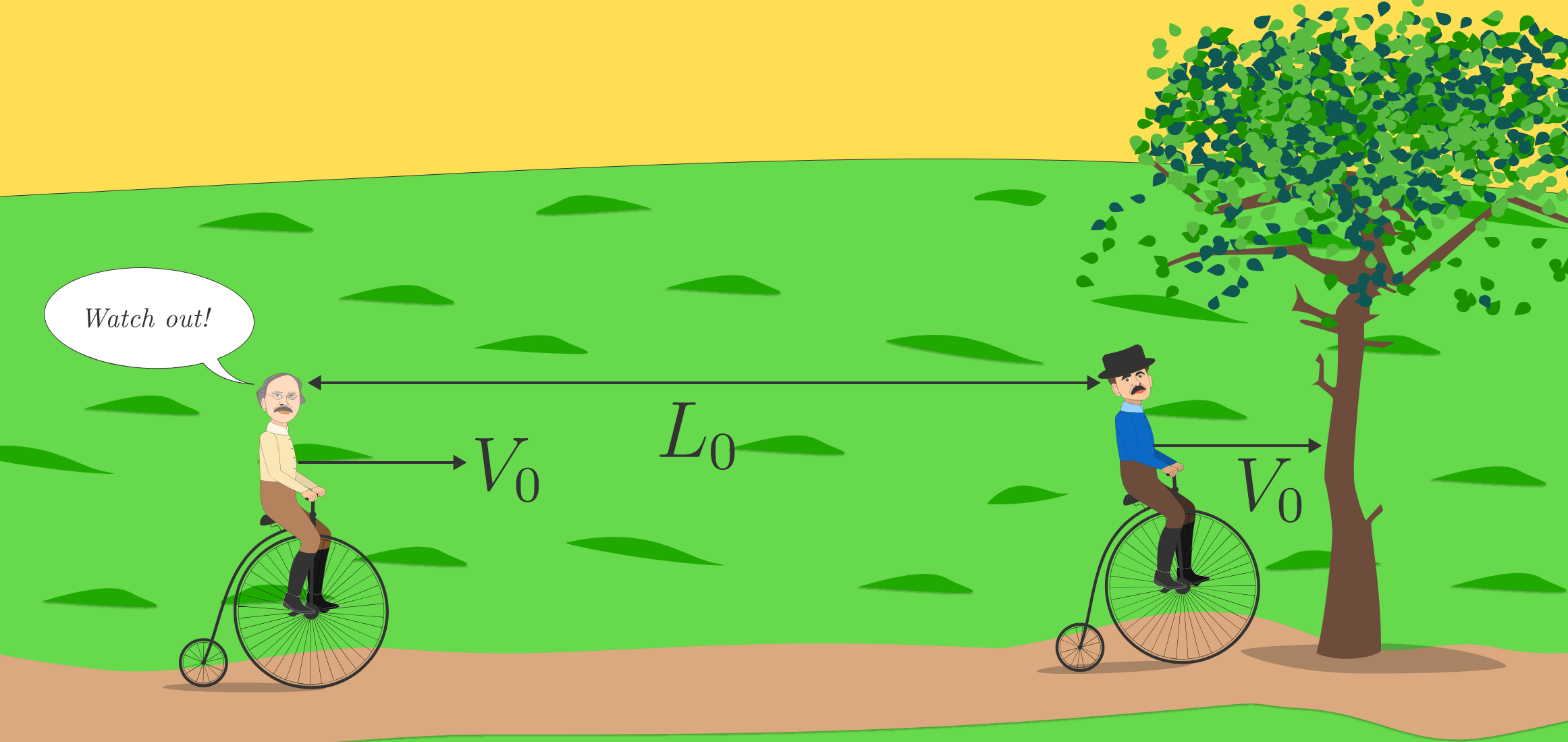Sound moves at a known speed of $c_\text{sound}$ relative to the air. If they were at rest in the air's reference frame, the sound would take time $\displaystyle \Delta T_0 =\frac{L_0}{c_\textrm{sound}}$ to travel distance $L_0$.

However, they are both moving relative to the air with speed $V_0.$ How long does it take Michelson to hear Morley?

# The Mystery of the Luminiferous Aether

A few minutes later, Michelson and Morley are riding with speed $V_0$ parallel to each other, a distance of $L_0$ from each other.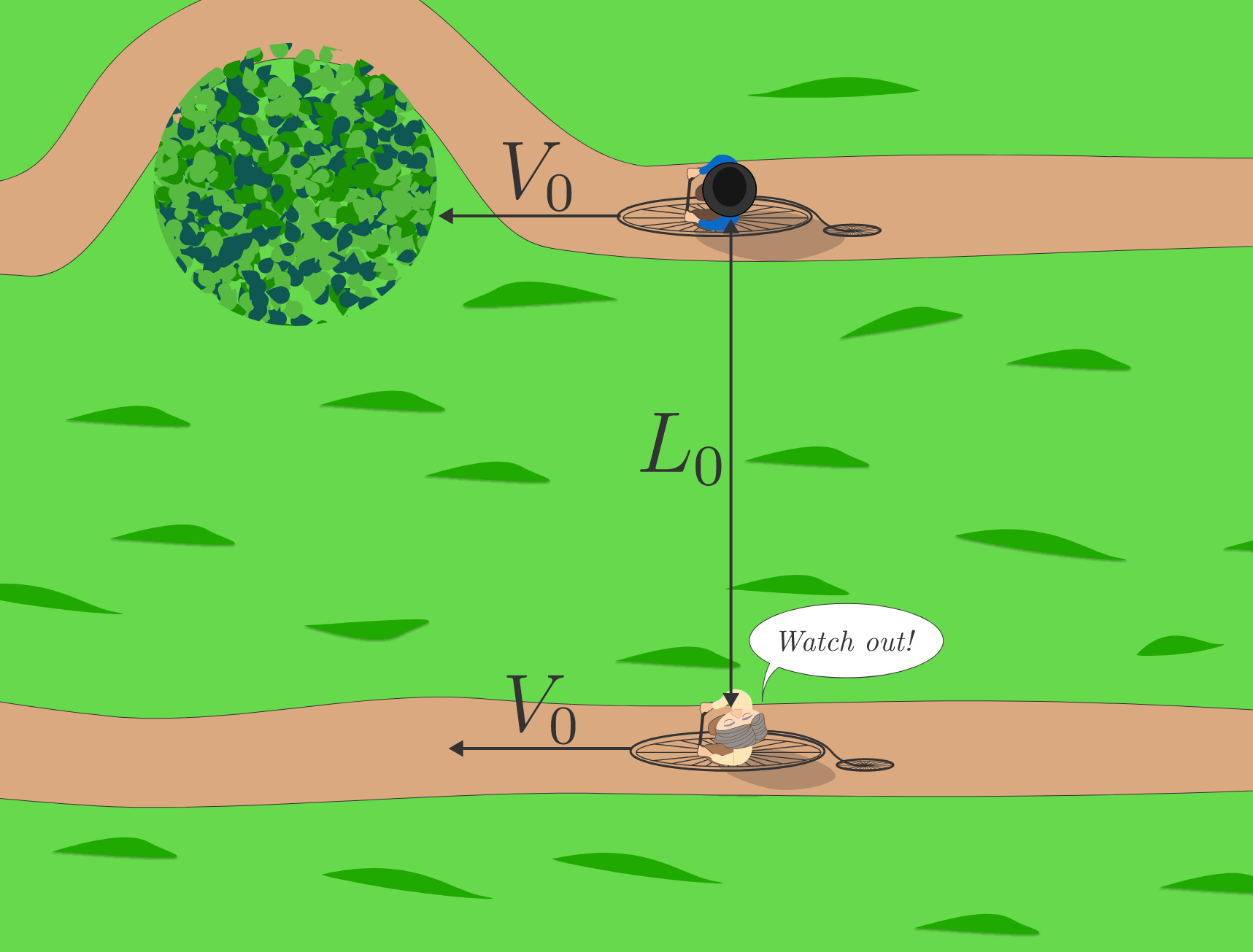Compared to $\Delta T_0,$ the propagation time when they are at rest relative to air, how long does it take Michelson to hear Morley?

Recall $\displaystyle \Delta T_0 = \frac{L_0}{c_\textrm{sound}}.$

# The Mystery of the Luminiferous Aether

Michelson and Morley's idea to prove (or disprove) the existence of aether was a straightforward extension of their recreational biking misadventures. If light moves at a speed $c$ through the aether, and Earth moves through the aether as it orbits the Sun, then light takes different amounts of time to propagate the same distance along perpendicular directions.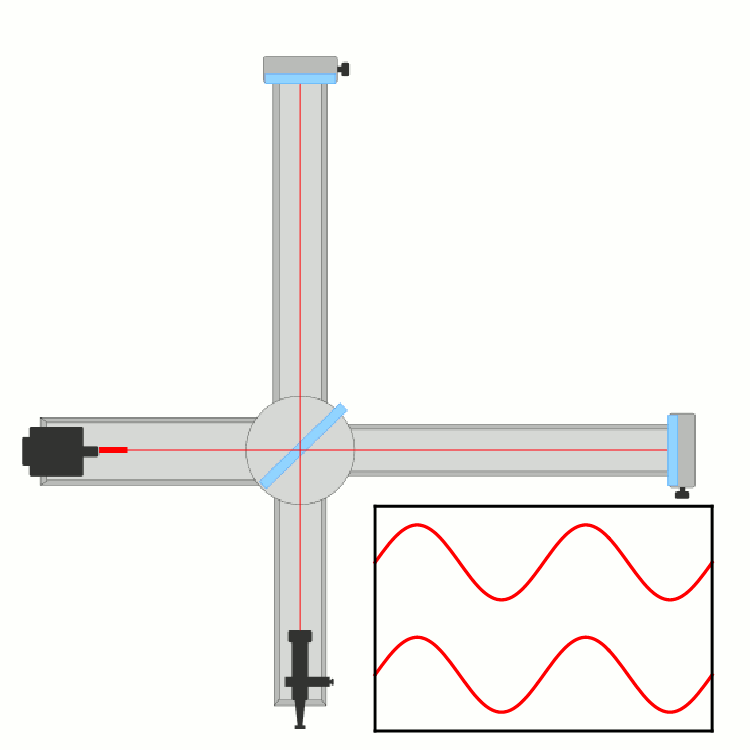So, they built a device called an interferometer with two identical, long arms at $90^\circ$ to one another and a coherent light source (the equivalent of a modern-day laser). They used a beam-splitter (a special kind of optical device made from metal-coated prisms) to divide the beam and send it toward mirrors at each end of each arm.

After they are reflected, the beams move back along the arms and are combined again before they enter a detector. If the propagation time along each arm is different, Michelson and Morley would detect interference (a mismatch between the waves' peaks and troughs) between the two beams—as long as the interferometer was moving relative to the aether.

# The Mystery of the Luminiferous Aether

Let's help Michelson and Morley predict the time difference between the arms, assuming the experiment moves with speed $v$ relative to the aether.

Imagine, for the sake of argument, we've managed to align one arm of the interferometer with $v$ as in the image below. The time $T_\text{parallel}$ for the beam to propagate from the beam-splitter to the mirror and back again depends on the setup's speed relative to the aether.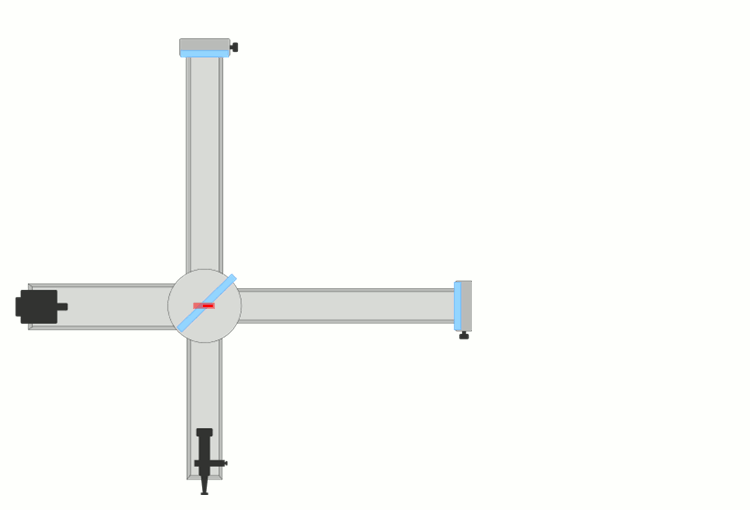Assuming the length of the interferometer's arm (from the beam-splitter to the mirror) is $L_0,$ which expression gives $T_\text{parallel}?$

Hint: Each leg of the trip will be the form of a fraction with different denominators. Find a common denominator and add the fractions.

# The Mystery of the Luminiferous Aether

We can compare this prediction to the time, $T_\textrm{perp},$ to travel back and forth along the other arm.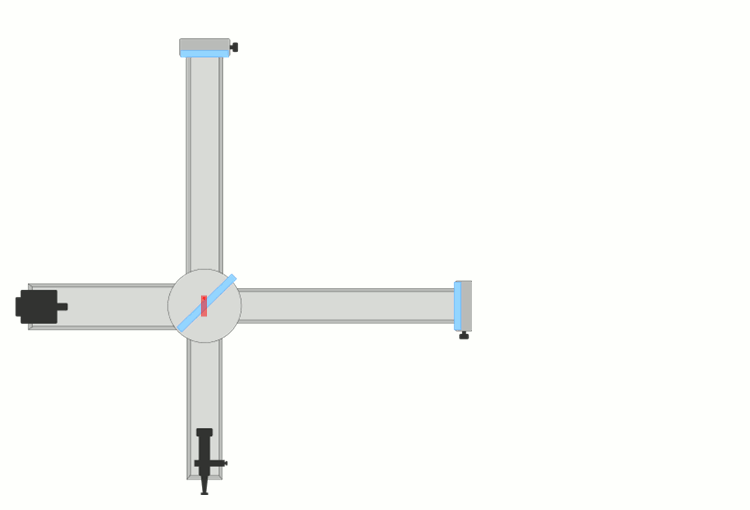When the interferometer is moving with speed $v$ relative to the aether, what is the propagation time along the arm perpendicular to the velocity?

# The Mystery of the Luminiferous Aether

Since $T_\textrm{parallel} \propto L_0$ and also $T_\textrm{perp} \propto L_0$, the predicted time difference between the arms is proportional to $L_0,$ too: $\Delta T = \big\lvert T_\textrm{parallel} - T_\textrm{perp} \big\rvert \propto L_0.$ Thus, the bigger your interferometer, the easier it is to detect the effect of moving through aether.

The interferometer Michelson and Morley used in their original experiment had $L_0=\SI{11}{\meter}.$ The split beam takes a different time to propagate along each arm. What is the time difference $\Delta T$ between the arms?

Assume that $v \sim \SI[per-mode=symbol]{30}{\kilo\meter\per\second},$ the orbital speed of Earth. At the time of this experiment, no one had any idea of how fast Earth was moving relative to the aether, but people agreed this was a reasonable lower limit. Also, use $c=\SI[per-mode=symbol]{3e5}{\kilo\meter\per\second}.$

# The Mystery of the Luminiferous Aether

Although this seems like a small time difference, it's long enough for the peaks and troughs of a light wave to become misaligned before they come back together in an interferometer the size of Michelson and Morley's.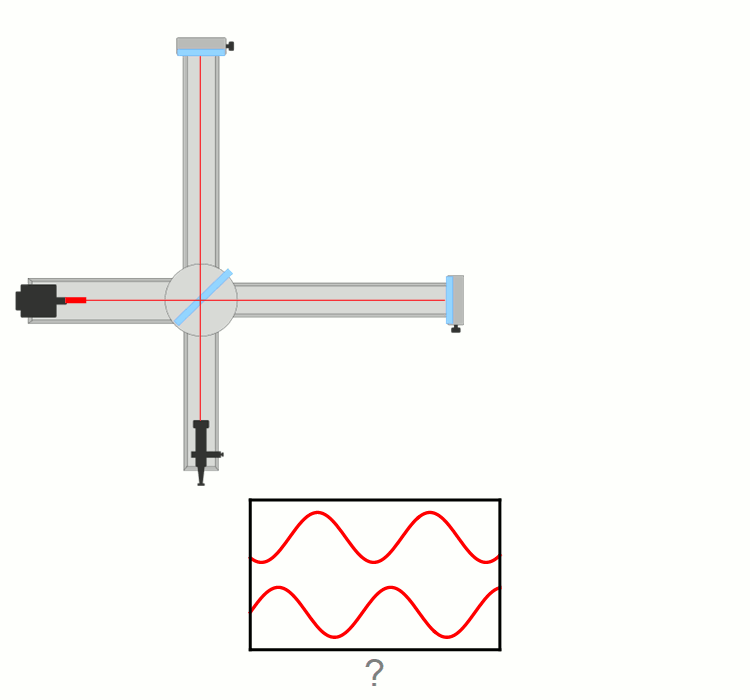Interference can be detected when the waves are misaligned by as little as a tenth of a wavelength. The wavelength of light is very short $\big($about $\lambda_\textrm{light} \approx \SI{700}{\nano\meter}=\SI{7e-7}{\meter}\big)$ and light travels fast, so the beams accrue a detectable wavelength difference $\Delta \lambda \gt 0.1 \lambda_\textrm{light}$ quickly.

The wavelength misalignment (also known as "decoherence") using the $\Delta T$ you calculated in the last problem is $\Delta \lambda = c\Delta T \approx \SI{1.2e-7}{\meter}.$ This is definitely above the detection threshold of Michelson and Morley's device.

# The Mystery of the Luminiferous Aether

If, indeed, Earth is moving through a mysterious luminiferous aether, then Michelson and Morley's interferometer was almost certain to detect its effect.

In this quiz, we derived an expression for the propagation time difference between the arms, $\Delta T,$ and we checked that our interferometer was the right size to detect such a minute effect.

However, regardless of how Michelson and Morley oriented their device relative to Earth's motion, they measured no time difference. The beams always recombined in such a way that no interference pattern was produced.

Which of the following could produce this result?

# The Mystery of the Luminiferous Aether

Michelson and Morley knew what they expected to see, and their experiment had the precision to see it. Their experiments convinced the scientific community near the turn of the $20^\text{th}$ century that both beams took exactly the same time to propagate along perpendicular arms of the interferometer.

For $18$ years, the community of physicists speculated that Earth shared a "special" rest frame with the undetectable aether. None of the speculations could be validated by experiment.

Then, in $1905,$ a young German patent clerk named Einstein posited that, indeed, the length of an interferometer's arms might actually depend on how fast Earth moves around the Sun, simultaneously killing the numerous theories of luminiferous aether and changing the way we view the universe completely.

# The Mystery of the Luminiferous Aether

×